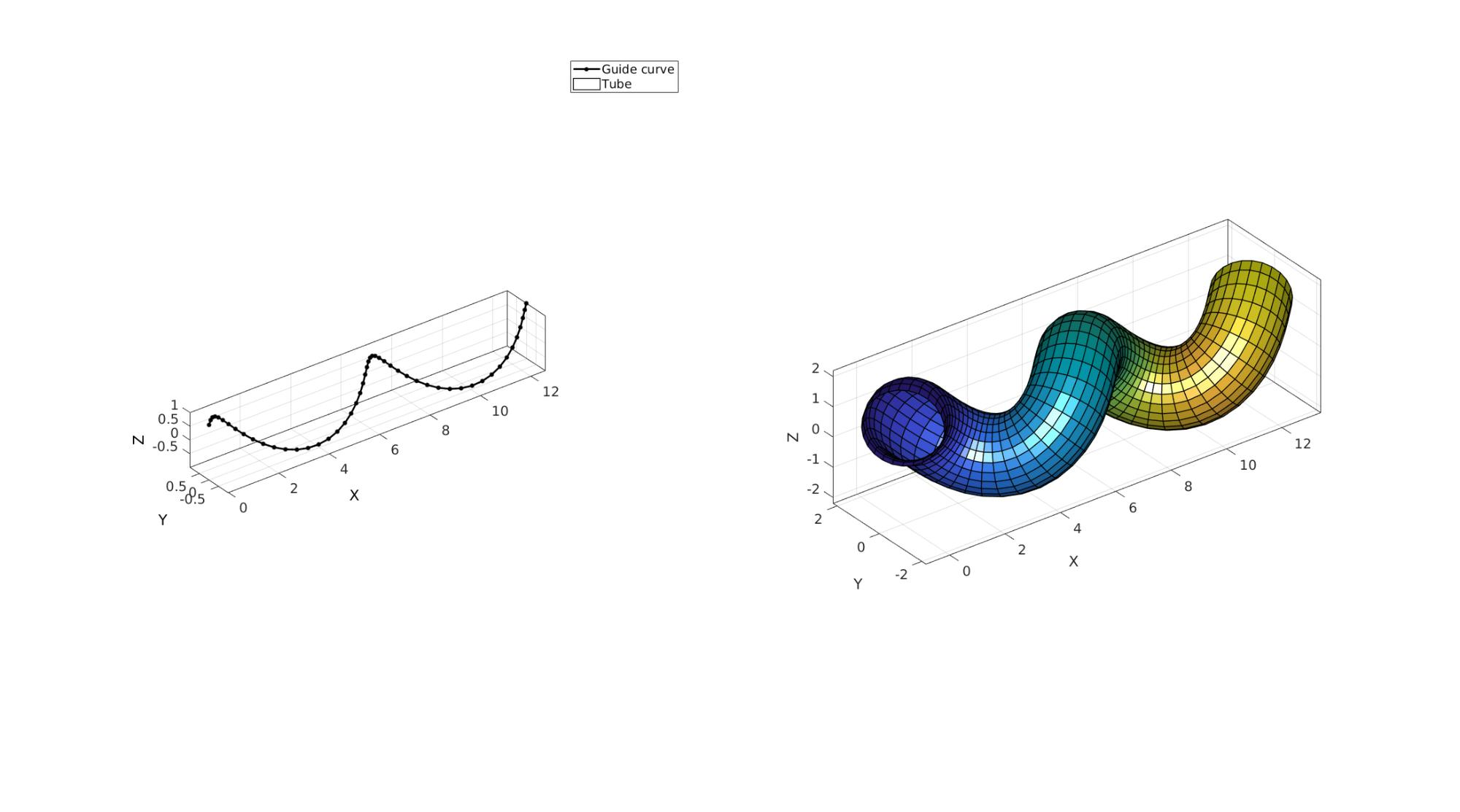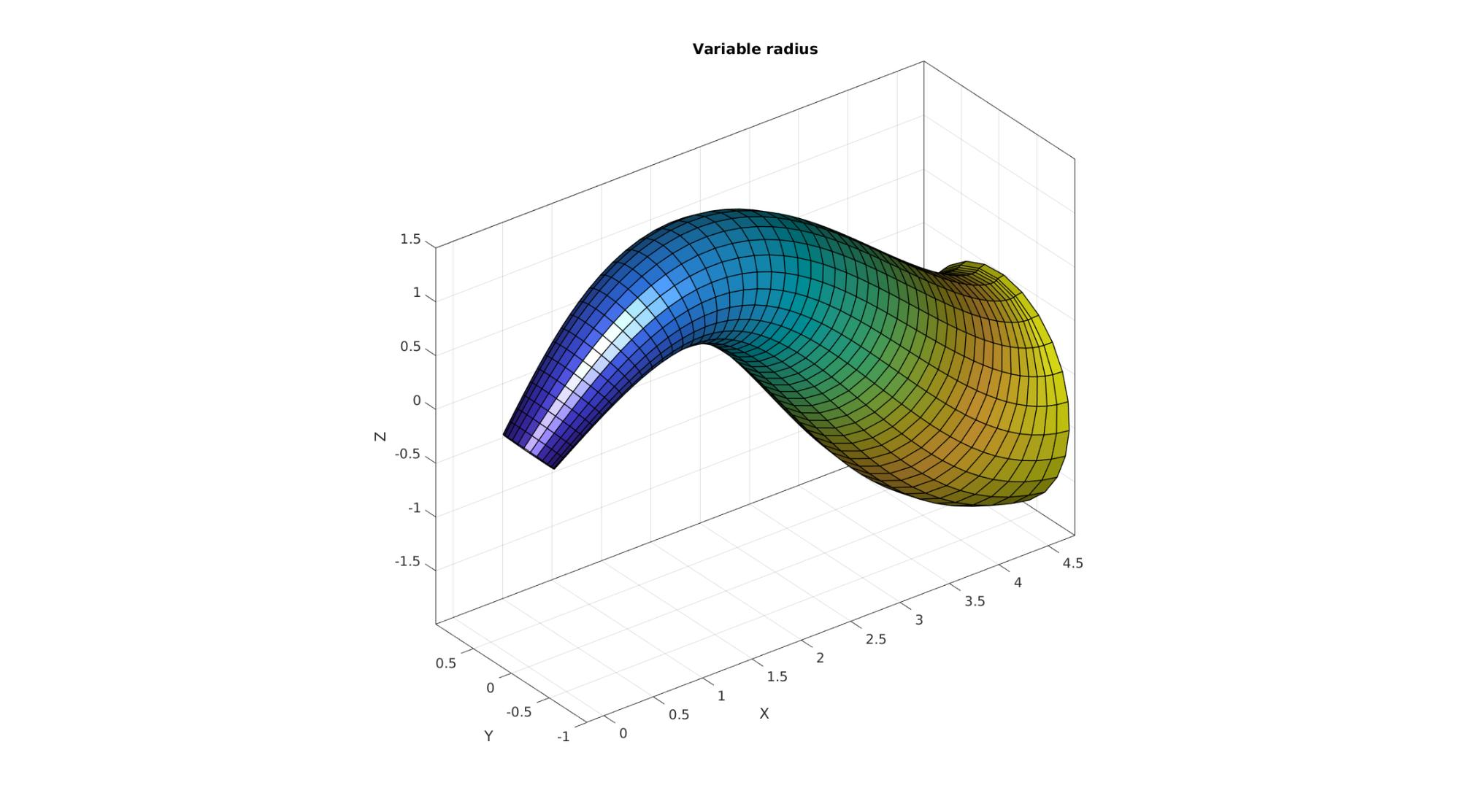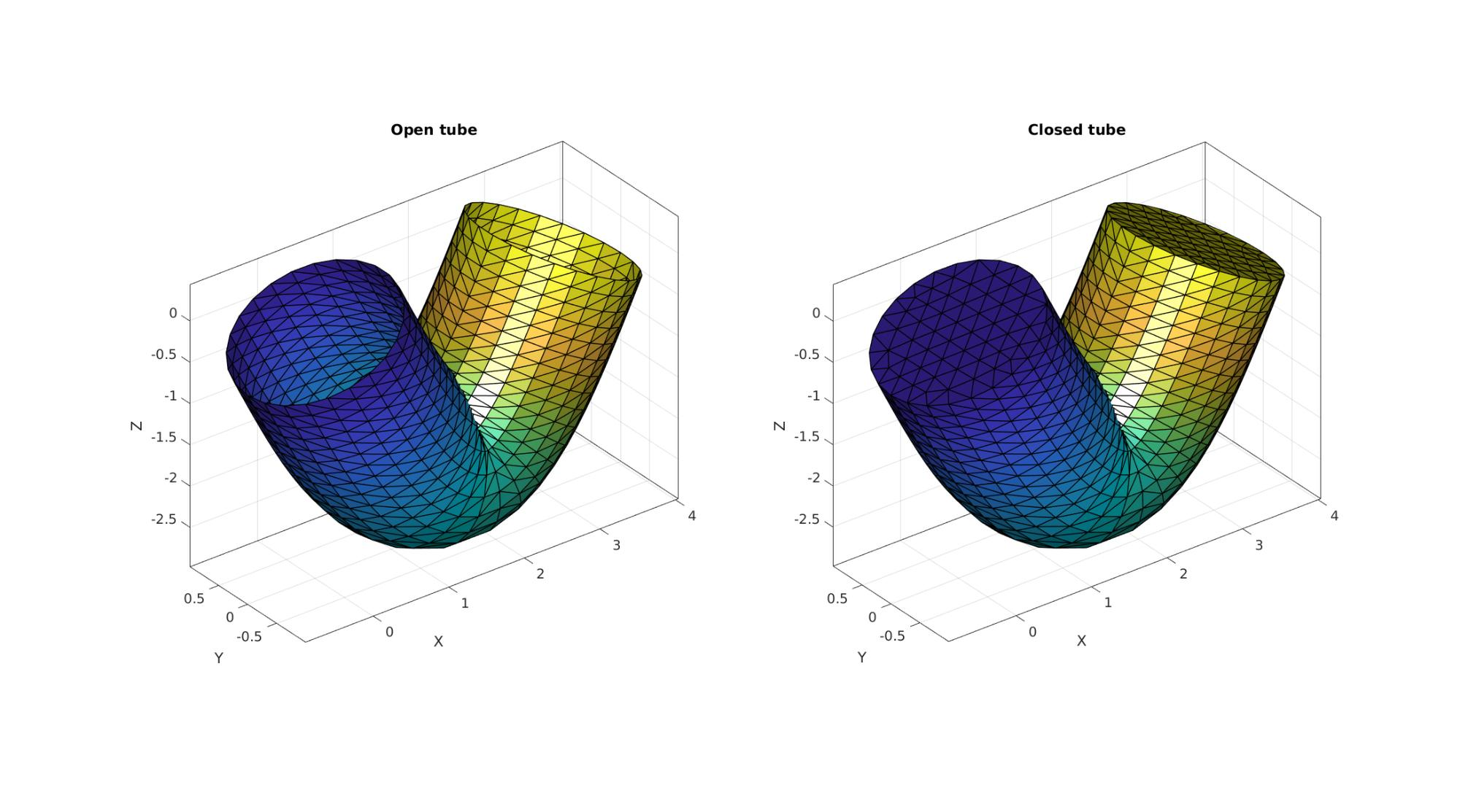# polyTube

Below is a demonstration of the features of the polyTube function

## Contents

```clear; close all; clc;
```

## Syntax

[Fs,Vs,Cs,Cs_rgb,Cs_d]=polyTube(Vg,optStruct);

## Description

The polyTube function can be used to

## Examples

Plot settings

```markerSize=15;
lineWidth=2;
fontSize=10;
```

## Example 1: Creating a swept tube

Creating example curve

```t=linspace(0,4*pi,50);
Vg=[ t(:) sin(t(:)) cos(t(:))];
Vg=evenlySampleCurve(Vg);
```

Create tube

```clear optStruct
optStruct.nr=25; %Number of points allong circumference
optStruct.fixOpt=0; %Option to fix self intersection

[Fs,Vs,Cs]=polyTube(Vg,optStruct);
```

Visualization

```cFigure;
subplot(1,2,1); hold on;
hp1=plotV(Vg,'k.-','MarkerSize',markerSize,'LineWidth',lineWidth);
axisGeom;

subplot(1,2,2); hold on;
hp2=gpatch(Fs,Vs,Cs,'k',1,1);

legend([hp1 hp2],{'Guide curve','Tube'});
gdrawnow;
```Creating example curve

```t=linspace(0,1.5*pi,50);
Vg=[t(:) zeros(size(t(:))) sin(t(:))];
Vg=evenlySampleCurve(Vg);
```

Create tube

```clear optStruct
optStruct.nr=25; %Number of points allong circumference
optStruct.fixOpt=0; %Option to fix self intersection

[Fs,Vs,Cs]=polyTube(Vg,optStruct);
```

Visualization

```cFigure; hold on;
gpatch(Fs,Vs,Cs,'k',1,1);
gdrawnow;
```## Example 3: Triangulated surface output and closed ends

Creating example curve

```r=linspace(0.25,1,30)'; %Defining radius allong curve
t=linspace(0,pi,30);
Vg=[t(:) zeros(size(t(:))) -2*sin(t(:))];
Vg=evenlySampleCurve(Vg);
```

Create tube with open ends

```clear optStruct
optStruct.r=1;
optStruct.nr=25;
optStruct.patchType='tri';
optStruct.closeOpt=0;
[Fs1,Vs1,Cs1]=polyTube(Vg,optStruct);
```

Create tube with closed ends

```clear optStruct
optStruct.r=1;
optStruct.nr=25;
optStruct.patchType='tri';
optStruct.closeOpt=1;
[Fs2,Vs2,Cs2]=polyTube(Vg,optStruct);
```

Visualization

```cFigure;
subplot(1,2,1); hold on;
title('Open tube')
gpatch(Fs1,Vs1,Cs1,'k',1,1);

subplot(1,2,2); hold on;
title('Closed tube')
gpatch(Fs2,Vs2,Cs2,'k',1,1);

gdrawnow;
```## Example 4: Using the fix option to attempt to fix self intersections

Creating example curve

```t=linspace(0,pi,30);
Vg=[t(:) 2*sin(t(:)) zeros(size(t(:))) ];
Vg=evenlySampleCurve(Vg);
```

Create tube without self-intersection fix

```clear optStruct
optStruct.r=1.2;
optStruct.nr=25;
optStruct.fixOpt=0;
[Fs1,Vs1,Cs1]=polyTube(Vg,optStruct);
```

Create tube with self-intersection fix

```clear optStruct
optStruct.r=1.2;
optStruct.nr=25;
optStruct.fixOpt=1;
[Fs2,Vs2,Cs2]=polyTube(Vg,optStruct);
```

Visualization

```cFigure;
subplot(1,2,1); hold on;
title('Self intersecting tube')
gpatch(Fs1,Vs1,'rw','k',0.5,2);
view(2);

subplot(1,2,2); hold on;
title('Fixed tube')
gpatch(Fs2,Vs2,'gw','k',1,2);
view(2);

gdrawnow;
```GIBBON www.gibboncode.org

Kevin Mattheus Moerman, [email protected]

GIBBON footer text

GIBBON: The Geometry and Image-based Bioengineering add-On. A toolbox for image segmentation, image-based modeling, meshing, and finite element analysis.

Copyright (C) 2006-2020 Kevin Mattheus Moerman

This program is free software: you can redistribute it and/or modify it under the terms of the GNU General Public License as published by the Free Software Foundation, either version 3 of the License, or (at your option) any later version.

This program is distributed in the hope that it will be useful, but WITHOUT ANY WARRANTY; without even the implied warranty of MERCHANTABILITY or FITNESS FOR A PARTICULAR PURPOSE. See the GNU General Public License for more details.

You should have received a copy of the GNU General Public License along with this program. If not, see http://www.gnu.org/licenses/.